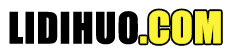Python数据处理
Python数据可视化
Python统计分析# Python数据操作

Python数据操作详细操作教程
Python主要通过 PandasNumpy这两个库来处理各种格式的数据。 我们已经在前面的章节中看到了这两个库的重要特征。 在本章中，我们将看到每个库中关于如何操作数据的一些基本示例。

## Numpy中的数据操作

NumPy中定义的最重要的对象是名为ndarray的N维数组类型。 它描述了相同类型的项目的集合。 可以使用从零开始的索引来访问集合中的项目。 ndarray类的一个实例可以通过本教程稍后介绍的不同阵列创建例程来构建。 基本的ndarray是使用NumPy中的数组函数创建的，如下所示 -
numpy.array

import numpy as np
a = np.array([[1, 2], [3, 4]])
print (a)

[[1, 2]
[3, 4]]

# minimum dimensions
import numpy as np
a = np.array([1, 2, 3,4,5], ndmin = 2)
print (a)

[[1, 2, 3, 4, 5]]

# dtype parameter
import numpy as np
a = np.array([1, 2, 3], dtype = complex)
print (a)

[ 1.+0.j, 2.+0.j, 3.+0.j]

## Pandas数据操作

Pandas 通过数列( Series)，数据框和面板处理数据。 我们将看到一些例子。
Pandas数列Series是一维标签数组，能够容纳任何类型的数据(整数，字符串，浮点数，python对象等)。 轴标签统称为索引。 Pandas系列可以使用以下构造函数创建 -
pandas.Series( data, index, dtype, copy)

# Filename : example.py
# Copyright : 2020 By Lidihuo
# Author by : www.lidihuo.com
# Date : 2020-08-22
#import the pandas library and aliasing as pd
import pandas as pd
import numpy as np
data = np.array(['a','b','c','d'])
s = pd.Series(data)
print (s)

0 a
1 b
2 c
3 d
dtype: object
Pandas数据帧

pandas.DataFrame( data, index, columns, dtype, copy)

# Filename : example.py
# Copyright : 2020 By Lidihuo
# Author by : www.lidihuo.com
# Date : 2020-08-22
import pandas as pd
data = {'Name':['Tom', 'Jack', 'Steve', 'Ricky'],'Age':[28,34,29,42]}
df = pd.DataFrame(data, index=['rank1','rank2','rank3','rank4'])
print df

Age Name
rank1 28 Tom
rank2 34 Jack
rank3 29 Steve
rank4 42 Ricky
Pandas面板

pandas.Panel(data, items, major_axis, minor_axis, dtype, copy)

# Filename : example.py
# Copyright : 2020 By Lidihuo
# Author by : www.lidihuo.com
# Date : 2020-08-22
#creating an empty panel
import pandas as pd
import numpy as np
data = {'Item1' : pd.DataFrame(np.random.randn(4, 3)),
'Item2' : pd.DataFrame(np.random.randn(4, 2))}
p = pd.Panel(data)
print (p)

<class 'pandas.core.panel.Panel'>
Dimensions: 2 (items) x 4 (major_axis) x 5 (minor_axis)
Items axis: 0 to 1
Major_axis axis: 0 to 3
Minor_axis axis: 0 to 4